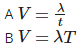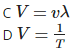Courses

# Sound (Test -1) - Class 9 Science, 21-02-2018

## 30 Questions MCQ Test | Sound (Test -1) - Class 9 Science, 21-02-2018

Description
This mock test of Sound (Test -1) - Class 9 Science, 21-02-2018 for Class 9 helps you for every Class 9 entrance exam. This contains 30 Multiple Choice Questions for Class 9 Sound (Test -1) - Class 9 Science, 21-02-2018 (mcq) to study with solutions a complete question bank. The solved questions answers in this Sound (Test -1) - Class 9 Science, 21-02-2018 quiz give you a good mix of easy questions and tough questions. Class 9 students definitely take this Sound (Test -1) - Class 9 Science, 21-02-2018 exercise for a better result in the exam. You can find other Sound (Test -1) - Class 9 Science, 21-02-2018 extra questions, long questions & short questions for Class 9 on EduRev as well by searching above.
QUESTION: 1

### Crests and troughs are formed in

Solution:

Crests and troughs are formed in transverse wave. The upward movement from mean position is called crest and downward movement is called troughs.

QUESTION: 2

### Statement A: Speed of sound is same at different temperature Statement B: Loudness is measured in decibels. Which of the two statements is true?

Solution:

Speed of sound is different at different temperature. With increase in temperature, speed of sound increases.

The loudness of sound is measured in units called decibels (dB). A decibel unit expresses the relative intensity of sounds on a scale from zero for the average least perceptible sound to about 100 dB, which is near the level most people find uncomfortably loud.

QUESTION: 3

### Match the following with correct response.  (1) Speed of sound in Aluminium  (2) Speed of sound in water  (3) Speed of sound in air at 25∘C (4) Speed of sound in oxygen gas  (A) 328 m/s  (B) 1520 m/s  (C) 6420 m/s  (D) 347 m/s

Solution:
QUESTION: 4

Principle of reflection of sound is used in

Solution:

The principle of reflection of sound is used in megaphone, sound boards and stethoscope and other instruments.

QUESTION: 5

X – rays, light rays are electromagnetic waves is a

Solution:

X-rays are a form of electromagnetic radiation, as are radio waves, infrared radiation, visible light, ultraviolet radiation and microwaves.These waves do not require material medium for its propagation.

QUESTION: 6

Frequency of infrasonic waves is ____________ than the frequency of ultrasound waves.

Solution:

Frequency of infrasonic waves is less than the frequency of ultrasound waves.
Frequency of infrasonic waves are less than 20Hz whereas frequency of ultrasonic waves are greater than 20,000Hz.

QUESTION: 7

Which of the following is correct-Solution:
QUESTION: 8

Match the following with correct response.
(1) Echo
(2) Reverberation
(3) SONAR
(4) Sound board

(A) Bouncing back of sound
(C) Persistence of sound due to repeated reflection
(D) Concave surface

Solution:

An echo is the repitition of the original sound heard after the sound is reflected from a distant,dense and rigid object.

When a number of echoes of the original sound are heard,each echo being fainter than the preceding one,such multiple echoes are called reververations.

SONAR (Sound Navigation and Ranging): Sonar is a device which is used to find the depth of the sea,or to locate underwater objects like enemy's submarine,sunken ships,shoal of fish,etc.

A sound board is a concave board (curved board) that is placed behind the speaker. The speaker is made to stand at the focus of the concave sound board. This setup solves the problem of interference of the sound waves with the large walls of the hall or auditorium.

QUESTION: 9

Sound wave is a

Solution:

Sound wave is a longitudinal wave as the wave particles of sound travel parallel to the direction of motion by forming compression and rarefaction.

QUESTION: 10

The velocity, wavelength and time period of a wave are related as

Solution:

Wave velocity =frequency×wavelength, frequencyfrequency×wavelength, frequency= 1/time–period. So, wave velocity = wavelength/ time–period.

QUESTION: 11

“Due to the high power of ultrasound waves. It is used for breaking and cutting objects”
The above statement is

Solution:

Ultrasound waves vibrate at frequency higher than 20,000Hz. Ultrasonic waves are directed towards kidneys.These waves with such a high vibration break the stones into tiny pieces so they can pass out of the kidney with the urine.

QUESTION: 12

Match the following with correct response.
(1) Audible range
(2) Infrasonic
(3) Ultrasonic
(4) Supersonic

(A) Less than 20 Hz
(B) Speed greater than sound in air
(C) More than 20,000 Hz
(D) 20 Hz to 20,000 Hz

Solution:

The sound whose frequency lies between 20 Hz and 20000 Hz which we are able to hear is called audible sound.

Inaudible sounds having frequency less than 20 Hz are known as infrasonic sounds.

Inaudible sounds having frequecy more than 20000 Hz are known as ultrasonic sounds.

When a body is travelling at a speed greater than the speed of sound,we say that the body is travelling at a supersonic speed.

QUESTION: 13

The maximum displacement of the particles of a medium from their mean position is called the

Solution:

The maximum displacement of the particles of a mediumfrom their mean position during the propagation of a wave is called the amplitude of the wave. Loudness of sound depends upon amplitude.

QUESTION: 14

The upper limit of frequency of sound waves audible to human beings is

Solution:

The sound whose frequency lies between 20 Hz and 20000 Hz which human beings are able to hear is called audible sound. So,the audible range of human ear is 20-20000 Hz.

QUESTION: 15

Statement A: Human beings are ultrasound deaf
Statement B: Ultrasonic waves can travel easily in solid, liquid and gases.
Which of the two statements is true?

Solution:

Human beings are ultrasound deaf as humans are not able to hear frequency of sound above 20,000 Hz.

Ultrasound travels through various media including gases, liquids and solids, but cannot travel through a vacuum. The speed of sound varies by the medium it travels through. Sound is likely to travel faster through solids, followed by liquids and gases.

QUESTION: 16

An echo is heard when-
(A) Minimum distance is 17.2 m
(B) Minimum time gap between two sounds is 1 / 10th of second
(C) Minimum distance is 18 m
(D) Minimum time gap between two sounds is 1 / 10th of second

Solution:

It has been found that the sensation of sound persists in our ears for 0.1 sec or one-tenth of a second,after the original sound dies off.

By further calculation,it is found that to hear an echo,the minimum distance from the sound-reflecting surface must be 17.2 m

QUESTION: 17

Which wave property determines loudness?

Solution:

Loudness of sound depends upon amplitude,more the amplitude of a wave, more is the loudness produced.Amplitude is the maximum displacement of a particle from its mean position.

QUESTION: 18

The distance between a consecutive crest and trough is x. The wavelength of the wave is

Solution:

Wavelength is the distance between two consecutive crest or trough in case of transverse wave. Here, distance between the consecutive crest and trough is x, so, wavelength will be 2x.

QUESTION: 19

Match the following with correct response.
(1) Laws of reflection of sound
(2) An application of multiple reflection of sound
(3) Ultrasound
(4) SONAR

(A) Megaphone
(B) Measures Depth of the sea
(C) To monitor foetus inside mother’s womb
(D) Angle of reflection is equal to angle of incidence

Solution:

According to the lwas of reflection of sound, the angle of incidence is equal to the angle of reflection of sound.

When a person speaks into the narrow end of the megaphone tube, the sound waves produced by his voice are prevented from spreading by successive reflections from the wider end of the megaphone tube.

Ultra-sonography uses ultrasounds for the examination of the foetus during pregnancy.The technique is used to monitor the growth,development and well being of the foetus.

SONAR (Sound Navigation and Ranging): Sonar is a device which is used to find the depth of a sea,or to locate underwater objects like enemy's submarine ,sunken ships,shoal of fish,etc.

QUESTION: 20

The eardrum is a

Solution:

The eardrum is a stretched membranous structure at the junction of outer ear and middle ear. Vibration of eardrum causes production of sound that we hear. It is also known as tympanic membrane.

QUESTION: 21

A source produces 20 crests and 20 troughs in 0.2 second. Find the frequency of the wave.

Solution:

One crest and a trough make a single wavelength.

Therefore,according to the question,20 wavelenghts are made.

Therefore, time = 0.2 sec

Time period=0.2/20 = 0.01 second.

Frequency = 1/time period = 1/0.01 = 100 Hz.

QUESTION: 22

Children can hear sound of frequency up to

Solution:

Children under the age of five can hear sound  up to 25,000Hz  which is more than normal adult hearing range. As people grow older their ears become less sensitive to higher frequency.

QUESTION: 23

Ultra sound is produced by
(A) Tortoise
(B) Bats
(C) Dolphins
(D) Dogs

Solution:

Animals like dolphins,bats and tortoises produce sounds of frequency higher than 20000 Hz known as ultrasonic sounds.

QUESTION: 24

Cochlea, semicircular canals and the auditory nerve are associated with

Solution:

Cochlea, semicircular canals and auditory nerve are associated with inner ear.The internal ear contains colchea.The vibrating bones pass their vibrations to the colchea.The sensitive hair cells present in the colchea respond to the sound vibrations recieved and change them into nerve impulses.These impulses are then carried by the auditory nerves to the brain.

QUESTION: 25

“Wave motion shifts mass from one region to another” The above statement is

Solution:

Wave motion shifts wave energy from one region to another region. Mass is not transferred during wave motion.

QUESTION: 26

A wave moves a distance of 10 m in 0.05 second. Find the wavelength of the wave if frequency is 200 Hz?

Solution:

We know that, velocity = distance travelled /time taken

=10 m/0.05 s

= 200m/s.

Now,

Wavelength = wave speed / frequency

⟹200 ms−1/200Hz = 1m.

Therefore,the wavelength of the wave is 1 m.

QUESTION: 27

A bat hears the echo of its squeak after 0.1 second. How far is the obstacle from the bat? Speed of sound is 344 ms-1

Solution:

Speed of sound = 344ms-1.

Time taken to hear echo = 0.1s

⟹2×distance=speed×time.

⟹2d=344×0.1

⟹ 2×d=34.4

⟹d=17.2

Therefore,the bat is 17.2 m from its obstacle.

QUESTION: 28

Wave motion is a periodic ____________ produced by a vibrating body.

Solution:

Wave motion is a periodic disturbance in the medium produced by vibrating body. These disturbance moves into medium to produce sounds. Wave motion is also known as propagation of disturbances.

QUESTION: 29

Calculate the wavelength of a sound wave whose frequency is 820Hz and speed is 440m/s in a given medium.

Solution:

Wavelength= wave speed / frequency, wavelength=440ms−1440ms−1/840Hz = 0.53m.

QUESTION: 30

Statement A: Speeds greater than the speed of sound in air are called infrasonic speed.
Statement B: the amount of sound energy, passing each second through unit area is called intensity of sound.
Which of the above two statement is true

Solution:

Speed of greater than the speed of sound in air is called ultrasonic sound. The amount of sound energy passing each second through unit area is called intensity of sound.